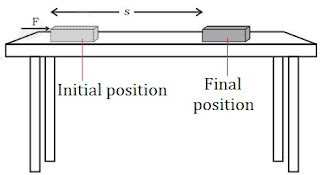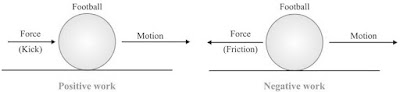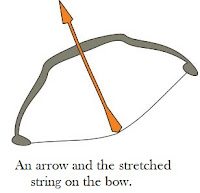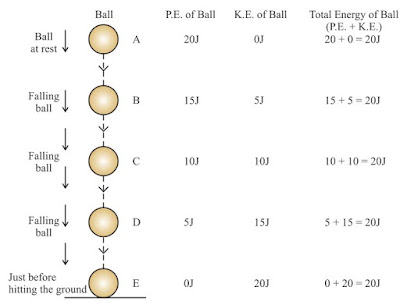#### Study Material and Notes of Ch 11 Work and Energy Class 9th Science

Topics in the Chapter

• Introduction
• Work
→ Condition for work to be done
→ Conditions when work is not done
→ Unit of Work
• Negative, positive and Zeo Work
• Energy
• Forms of Energy
→ Mechanical Energy
→ Kinetic Energy
• Potential Energy
• Transformation Energy
• Law of conservation Energy
• Free fall of a Body (Energy Conservation)
• Power
→ Unit of Power
• Commercial unit of Power
• Solved Numericals

Introduction

→ The energy for the ‘life processes’ comes from food. Activities like playing, singing, reading, writing, thinking, jumping, cycling and running requires energy.

Work

→ For doing work, energy is required.

• In animals, energy is supplied by food they eat.
• In machine, energy is supplied by fuel.

Why sometimes not much work is done inspite of working hard?

→ Reading, writing, drawing, thinking,analysing are all energy consuming. But in scientific manner, no work is done in above cases.

→ Example: A man is completely exhausted in trying to push a rock (wall), but work done is zero as wall is stationary.

→ A man standing still with heavy suitcase may be tired soon but he does no work in this situation as he is stationary.When force is applied on the wall, the wall doesn't move. Therefor, no work is done here.

• Work is said to be done when:

(i) a moving object comes to rest.
(ii) an object at rest starts moving.
(iii) velocity of an object changes.
(iv) shape of an object changes.

Scientific Conception of Work

→ Work is done when a force produces motion in a body.

→ Work is said to be done when a force is applied on a body and the body moves under the influence of force.

Condition of Work

(i) Force should be applied on the body.
(ii) Body should be displaced.

• Work is done when:

(i)  A cyclist is pedaling the cycle.(ii) A man is lifting load in upward or downward direction.

• Work is not done when:

(ii) A man is applying force on a big rock.

Work Done by a Fixed Force

→ Work done in moving a body is equal to the product of force and displacement of body in the direction of force.Work = Force × Displacement
W = F × S

→ Work is a scalar quantity.

Unit of Work

→ Unit of work is Newton metre or Joule.

→ When a force of 1 Newton moves a body through a distance of 1 metre in its own direction, then the work done is 1 Joule.

1 Joule = 1 Newton × 1 metre
1 J = 1 Nm• The amount of work done depends on the following factors:

(i) Magnitude of force: Greater the force, greater is the amount of work & vice-versa.

(ii) Displacement: Greater the displacement, greater is the amount of work & vice-versa.

Negative, Positive and Zero Work

→ Work done by a force can be positive, negative or zero.

(i) Work done is positive when a force acts in the direction of motion of the body.

Example: A child pulls a toy car with a string horizontally on the ground.

Here work done is positive.
W = F × S(ii) Work done is negative when a force acts opposite to the direction of motion of the body.

Example: When we kick a football lying on the ground, the force of our kick moves the football. Here direction of force applied & motion of football is same so work done is positive. But when football slows due to force of friction acting in a direction opposite to direction of motion of football, thus work done is negative.(iii) Work done is zero when a force acts at right angles to the direction of motion.

Example: The moon moves around the earth in circular path. Here force of gravitation acts on the moon at right angles to the direction of motion of the moon. So work done is zero.Note: If work is done against the direction of motion (gravity), then it is taken –ve.

Example: A coolie lifts a luggage of 15 kg from the ground and put it on his head 1.5 m above the ground. Calculate the work done by him on the luggage.

Solution

Mass of luggage (m) = 15 kg
Displacement (S) = 1.5 m
So, Work done (W) = F × S
= mg × S  [f = mg]
= 15 × 10 × 1.5 [g = 10 m/s2 = force of gravity]
= 225.0 kg m/s2
= 225 Nm = 225 J
Hence, work done = 225 J.

Energy

→ The capacity of doing work is known as energy.

(i) The sun is the biggest source of energy.
(ii) Most of the energy sources are derived from the sun.
(iii) Some energy is received from nucleus of atoms, interior of the earth and the tides.

→ The amount of energy possessed by a body is equal to the amount of work it can do.

→ Working body losses energy, body on which work is done gains energy.

→ Energy is a scalar quantity.

Unit: The SI unit of energy is Joule (J) and its bigger unit is kilo joule (kJ).
1 kJ = 1000 J

→ The energy required to do 1 Joule of work is called 1 Joule energy.

Forms of Energy

• Main forms of energy are:

(i) Kinetic energy
(ii) Potential energy
(iii) Heat energy
(iv) Chemical energy
(v) Electrical energy
(vi) Light energy
(vii) Sound energy
(viii) Nuclear energy

Mechanical energy

→ Sum of kinetic energy & potential energy of a body is called mechanical energy.

→ The energy possessed by a body on account of its motion or position is called mechanical energy.

Kinetic Energy

→ The energy of a body due to its motion is called kinetic energy.

• Examples of kinetic energy
→ A moving cricket ball
→ Running water
→ A moving bullet
→ Flowing wind
→ A moving car
→ A running athelete
→ A rolling stone→ Kinetic energy is directly proportional to mass and the square of velocity.

Formula for Kinetic Energy

→ If an object of mass ‘m’ moving with uniform velocity ‘u’, it is displaced through a distance ‘s’. Constant force ‘f’ acts on it in the direction of displacement. Its velocity changes from ‘u’ to ‘v’.

→ Then acceleration is ‘a’.
Work done, W = F × s  ....(i)
F = ma  ....(ii)

→ According to third equation of motion, relationship between u, v, s and a is as follows:
v2 - u2 = 2as
⇒ s = (v2 - u2)/2a .... (iii)

Now putting the value of f and s from (ii) and (iii) in equation (i),If u = 0 (when body starts moving from rest)
W = ½mv2
E K = ½mv2

Example: An object of mass 15 kg is moving with uniform velocity of 4 m/sec. What is the kinetic energy possessed by it?

Solution

Mass of the object (m) = 15 kg
Velocity of the object (v) = 4 m/s
EK = ½mv2
= ½ × 15 kg × 4 ms-1× 4 ms-1 = 120 J

→ The kinetic energy of the object is 120 J.

Potential Energy

→ The energy of a body due to its position or change in shape is known as potential energy.

• Examples:

(i) Water kept in dam : It can rotate turbine to generate electricity due to its position above the ground.

(ii) Wound up spring of a toy car : It possess potential energy which is released during unwinding of spring. Therefore, toy car moves.

(iii) Bent string of bow : Potential energy due to change of its shape (deformation) released in the form of kinetic energy while shooting an arrow.Factors affecting Potential Energy

(i) Potential Energy:
P. E. ∝ m
→ More the mass of body, greater is the potential energy and vice-versa.

(ii) Height above the ground:
P. E. ∝ h (Not depend on the path it follows)

→ Greater the height above the ground, greater is the P.E. and vice-versa.

(iii) Change in shape: Greater the stretching, twisting or bending, more is the potential energy.

Potential Energy of an Object on a Height

→ If a body of mass ‘m’ is raised to a height ‘h’ above the surface of the earth, the gravitational pull of the earth (m × g) acts in downward direction.

→ To lift the body, we have to do work against the force of gravity.

Thus, Work done (W) = Force × Displacement
⇒ W  = m × g × h = mgh

→ This work is stored in the body as potential energy (gravitational potential energy).
Thus, Potential energy, Ep = m × g × h
where,
g = acceleration due to gravity.Example: If a body of mass 10 kg is raised to a height of 6 m above the earth, calculate its potential energy.

Solution

Potential energy of the body = mgh
Mass of body  = 10 kg
Height above the earth = 6 m
Acceleration due to gravity = 10 m/s2
So, Ep = 10 × 10 × 6 = 600 J

Thus, potential energy of the body is 600 Joules.

Transformation of Energy

The change of one form of energy to another form of energy is known as transformation of energy.

Example:

(i) A stone on a certain height has entire potential energy. But when it starts moving downward, potential energy of stone goes on decreasing as height goes on decreasing but its kinetic energy goes on increasing as velocity of stone goes on increasing.

→ At the time stone reaches the ground, potential energy becomes zero and kinetic energy is maximum. Thus, its entire potential energy is transformed into kinetic energy.

(ii) At hydroelectric power house, the potential energy of water is transformed into kinetic energy and then into electrical energy.

(iii) At thermal power house, chemical energy of coal is changed into heat energy, which is futher converted into kinetic energy and electrical energy.

(iv) Plants use solar energy to make chemical energy in food by the process of photosynthesis.

Law of Conservation of Energy

→ Whenever energy changes from one form to another form, the total amount of energy remains constant.

→ “Energy can neither be created nor be destroyed.”

→ Although some energy may be wasted during conversion, but the total energy of the system remains the same.

Conservation of Energy during Free Fall of a Body

→ A ball of mass ‘m’ at a height ‘h’ has potential energy = mgh.

→ As ball falls downwards, height ‘h’ decreases, so the potential energy also decreases.

→ Kinetic energy at ‘h’ is zero but it is increasing during falling of ball.

→ The sum of potential energy & kinetic energy of the ball remains the same at every point during its fall.
½mv2 + mgh = Constant
⇒ Kinetic energy + Potential energy = ConstantRate of Doing Work (Power)

→ “Power is defined as the rate of energy consumption.”
Power (P) = Work done/Time Taken = W/t

where,
P = Power
W = Work done
t = Time taken

Unit of Power

→ SI unit of Power is Watt (W) = 1 Joule/second.
1 Watt (W) = 1Joule/1 second = 1J/1s

→ Power is one Watt when one Joule work is done in one second.

• Average Power = Total work done or total energy used/Total time taken

→  The power of an electrical appliance tells us the rate at which electrical energy is consumed by it.

Bigger unit of Power

→ Bigger unit of power is called Kilowatt or KW.
1 Kilowatt (KW) = 1000 Watt = 1000 W or 1000 J/s

Example: A body does 20 Joules of work in 5 seconds. What is its power?

Solution

Work done = 20 Joules
Time taken = 5 sec.

∵ Power = Work done/Time taken
P = 20 J/5 s
∴ Power  = 4 J/s = 4 W
Thus, power of the body is 4 Watts.

Commercial Unit of Energy

→ Joule is very small unit of energy and it is inconvenient to use it where a large quantity of energy is involved.

→ For commercial purpose, bigger unit of energy is Kilotwatt hour (KWh).

1 KWh: 1 KWh is the amount of energy consumed when an electric appliance having a power rating of 1 Kilowatt is used for 1 hour.

Relation between Kilowatt hour and Joule

→ 1 Kilowatt hour is the amount of energy consumed at the rate of 1 Kilowatt for 1 hour.
1 Kilowatt hour  = 1 Kilowatt for 1 hour
= 1000 Watt for 1 hour
= 1000 Watt × 3600 seconds (60 × 60 seconds = 1 hour)
= 36,00,000 Joules

∴ 1 KWh = 3.6 × 106 J = 1 unit

Example: A bulb of 60 Watt is used for 6 hrs. daily. How many units (KWh) of electrical energy are consumed?

Solution

Power of bulb  = 60 W = 60/1000 KW = 0.06 KW
t = 6 hours

Energy = Power × Time taken = 0.06 × 6 h
= 0.36 KWh = 0.36 units